Study Materials

# NCERT Solutions for Class 8th Mathematics

Page 1 of 6

## Chapter 2. Linear Equations in One Variable

### Exercise 2.1

Exercise 2.1

Solve the following equations.

1.  x - 2 = 7

Solution:

x - 2 = 7

x = 7 + 2

x = 9

2. y + 3 = 10

Solution:

y + 3 = 10

y = 10 - 3

y = 7

3. 6 = z + 2

Solution:

6 = z + 2

z = 6 - 2

z = 4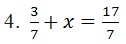Solution: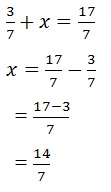5. 6x = 12

Solution:

x = 12/6

x = 2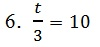Solution:

t = 3 × 10

t = 30

7. 2x + 3 = 18

Solution:

2x = 18 - 3

2x = 15

x = 15/2

x = 7.5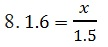Solution:

x = 1.6 × 1.5

x =  2.4

9. 7x - 9 = 16

Solution:

7x = 16 + 9

7x = 25

x = 25/7

x = 3.5

10. 14x - 8 = 13

Solution:

14x = 13 + 8

14x = 21

x = 21/14 = 3/2 = 1.5

11. 17 + 6p = 9

Solution:

6p = 9 - 17

6p = -8

p = -8/6 = - 1.3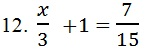Solution: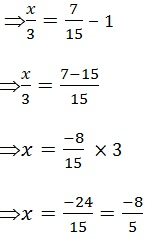Page 1 of 6

Chapter Contents: